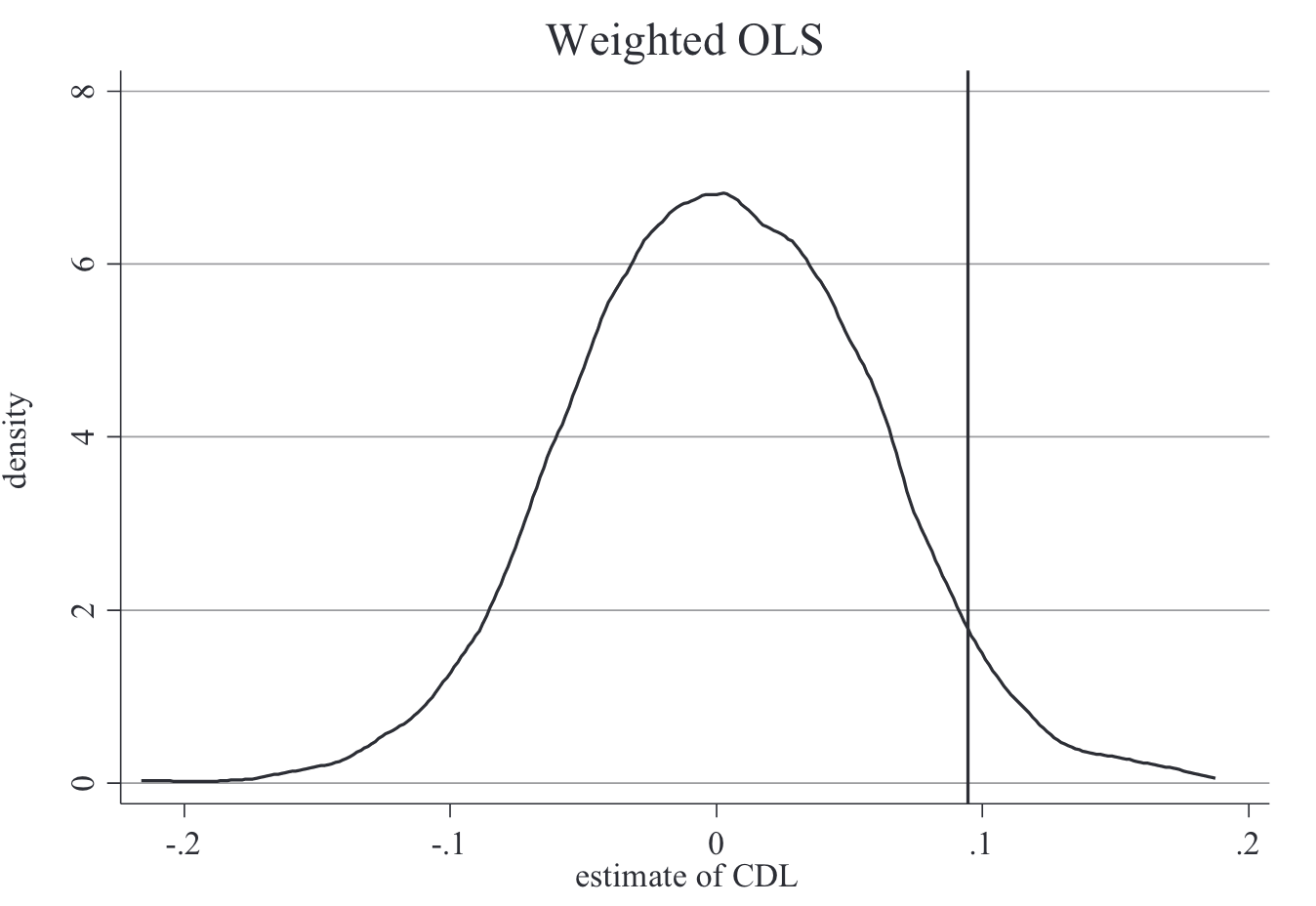# Difference-in-Differences

Series - Causal inference
Contents

The basic setting is: $$Y_{ist}=\alpha+\lambda_{s}+\lambda_{t}+\delta\times Treat_{s}\times Post_{t}+\beta X_{it}+\varepsilon_{it}$$

• $i$, $s$, and $t$ represent unit (e.g., person), group (e.g., state), and time (e.g., year).
• $Treat_{s}$ is a dummy and is 1 if $s$ belongs to the treatment group. $Post_{t}$ is a dummy and is 1 if $t$ is post-treatment.
• $\lambda_{s}$ and $\lambda_{t}$ are fixed effects for group and time
• $X_{it}$ is covariates.
• $\delta$ is the treatment effect to be estimated.

Don’t forget to cluster standard errors by group $s$ and time $t$!

One issue of the 2x2 model is that

• It doesn’t validate the parallel trend assumption
• It requires a single treatment model, while in practices groups are treated at different times
• many studies collect multiple periods of data before and after the treatment, while 2x2 model only has two periods.

Event study model is proposed to solve the issue. The setting is: $$Y_{ist}=\alpha+\lambda_{s}+\lambda_{t}+Treat_{s}\times \sum_{\tau=-q}^{-1} \gamma_{\tau}D_{\tau}+Treat_{s}\times \sum_{\tau=0}^{m} \delta_{\tau}D_{\tau}+\beta X_{it}+\varepsilon_{it}$$

• $i$, $s$, and $t$ represent unit (e.g., person), group (e.g., state), and time (e.g., year).
• $Treat_{s}$ is a dummy and is 1 if $s$ belongs to the treatment group. $D_{\tau}$ is a dummy and is 1 if current year belongs to the $\tau$’s leads/lags.
• $\lambda_{s}$ and $\lambda_{t}$ are fixed effects for group and time
• $X_{it}$ is covariates.
• $\gamma_{\tau}$ is the “pre-treatment parallel trends”. It’ll be zero if the parallel trends assumption is met.
• $\delta_{\tau}$ is the treatment effect to be estimated. It’s expected to be non-zero.

Ideally, the plot of $\gamma_{\tau}$ (the left part of the figure) and $\delta_{\tau}$ (the right part) should look like this:

What event study plots can NOT do?

The pre-treatment plot only shows the trends are parallel before the treatment. It can’t tell us if the dynamic remains the same after the treatment.

That is, it’s only an “approximation” of the parallel trend assumption.

Placebo falsification helps to mitigate the following two concerns:

• Alternative hypothesis. Placebo falsification can help to rule out alternative hypothesis. To do that, we keep the same treatment but replace the Y with alternative outcomes.
• The validity of the significance (p value). The common approach is randomization inference.
The key idea

“The reasoning goes that if we find, using our preferred research design, effects where there shouldn’t be, then maybe our original findings weren’t credible in the first place.”

In the example of minimal wage. We simply fit the same DD design using high wage employment as the outcome. If the coefficient on minimum wages is zero when using high wage worker employment as the outcome, but the coefficient on minimum wages for low wage workers is negative, then we have provided stronger evidence that complements the earlier analysis we did when on the low wage workers."

Another example is the “Medicare expansion - mortality” study (Cheng & Hoekstra, 2013)

More often used when there’s only one treatment group

Consider the following DD model (randomization inference doesn’t apply to event study model since it requires there’s only one treatment dummy) with a twoway standard error clustering: $$Y_{ist}=\alpha+\lambda_{s}+\lambda_{t}+\delta\times Treat_{s}\times Post_{t}+\beta X_{it}+\varepsilon_{it}$$ Cluster the standard errors on the state-year level simply assumes constant correlation at the state-year level, yet there may still be severe correlation within a state-year cell. To do a randomization inference, one:

• first, randomly choose a different treatment date. For example, move the treatment year backward for 1, 2, 3, etc. years.
• second, randomly assign treatment to states. For example, if in the original data 30% are treated and 70% are non-treated, then we randomly (without replacement) sample 30% of the data as the new treated units.
• In total, there’re N-new-date-assignment x N-new-state-assignment combinations. One usually randomly draw a few from it.
• Plot the p-value distribution of these placebo estimates and compare it with the “real” one. An example from (Cheng & Hoekstra, 2013):width=400

When using twoway fixed effects model to estimate the treatment, it was nothing more than an “adding up” of all possible 2×2s weighted by group shares and treatment variance. The Bacon decomposition reveals a fatal issue of DD: The coefficients on the static twoway fixed-effects leads and lags will be unintelligible if there is heterogeneity in treatment effects over time. In this sense, we are back in the world that Goodman-Bacon (2019) revealed, in which heterogeneity treatment effect biases create real challenges for the DD design using twoway fixed effects.

How to check if we have a severe late-to-early heterogeneity issue using the Bacon decomposition?

In R, there’s a package called bacondecomp. See below for how to interpret its results: